# Plot Page - Bar Charts

The bar chart plot properties Plot page contains the options to change the data file and axes; set data used in the plot; add a new plot to the graph; and set bar chart properties. To view and edit bar chart plot properties, click on the bar chart plot in the plot window or Object Manager to select it. Then, click on the Plot tab in the Property Manager. The properties available will be specific to the type of plot.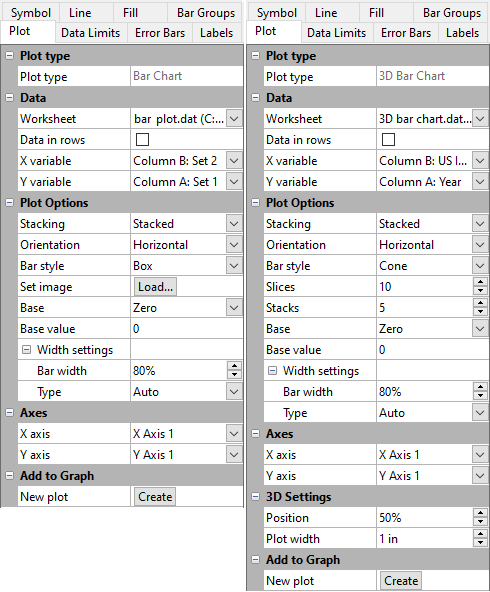Bar chart and 3D bar chart Plot properties examples

## Plot Type

The Plot type property displays the type of plot.

## Data

### Worksheet

Click the existing data file path and file name next to Worksheet to select a different data file for the plot. Click on a currently open worksheet or click the Browse option to navigate to a worksheet that is not yet open. Select a different data file in the Open Worksheet dialog, and click the Open button to change the data. The plot automatically updates.

NOTE: The worksheet columns are retained after changing the worksheet. If any of the column options are blank after changing the Worksheet, click on the blank option and select the desired column. A blank next to column means that the column does not exist in the new worksheet.

### Data in Rows

Select the Data in rows option if your data file is formatted where each row includes one variable. When the Data in rows option is selected, all plot properties that require data values are specified by selecting a data row. This includes variables such as X and Y, data labels, worksheet ranges, etc.  Properties that list column letters will update to list row numbers. When switching from plotting data in columns to plotting data in rows, Grapher may not be able to select the correct rows automatically. Verify the correct rows are selected.

The property descriptions below refer to data columns, but when Data in rows is selected the property lists will include rows.

### Variables

Click on the column name next to the X variable, Y variable, and Z variable fields to change the columns used to create the plot. In the list that appears, select the new column. If the first worksheet row contains header information, the header information appears next to the column letter.

Aside from the columns containing data there are two other options, row number and sequence number. These options are available in the list that appears before the first column.

• Selecting the Row number option plots the axis with the row numbers used in the Worksheet rows section. For example, if the first row is number two, the axis begins at a value of two.

• The Sequence number option plots the data according to the total number of rows used. The sequence number always begins with the number one regardless of which rows are selected in the Worksheet rows group. Blank lines between rows are ignored when calculating the value. The sequence number increments by one when rows are adjacent or when rows are skipped.

When changing the X variable, Y variable, or Z variable to date/time data from numeric data, the Use date/time format option for the corresponding axis Tick Labels will automatically be checked to match the new data. When changing the X variable, Y variable, or Z variable to numeric data from date/time data, the Use date/time format option for the corresponding axis Tick Labels will automatically be unchecked to match the new data.

NOTE: The worksheet columns are retained after changing the worksheet. If any of the column options are blank after changing the Worksheet, click on the blank option and select the desired column. A blank next to column means that the column does not exist in the new worksheet.

## Plot Options

### Plotting Multiple Variables

If multiple variables are plotted, the bars can be stacked or adjacent. Change the Stacking option to either Stacked or Adjacent. This setting affects all bar charts in a single bar chart group when multiple bar charts are plotted on one graph.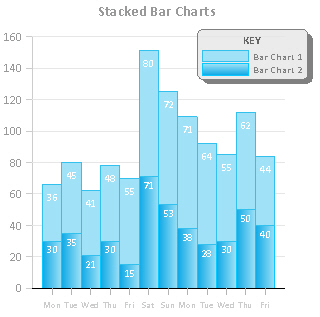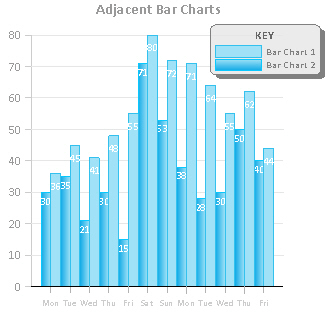These bars are stacked. The second bar is placed starting at the top of the first bar. These bars are adjacent. The bars are located side by side.

The Adjacent option draws variables as a group of bars. The group of bars for each X value are centered on the X value data value.

You can set the amount of overlap of the adjacent bars by typing a new number into the Bar overlap box. An overlap of zero means the bars in the same X value are touching. An overlap of greater than 0 means that the bars are overlapping. Using negative numbers in the Bar overlap field creates gaps between the bars within an X value.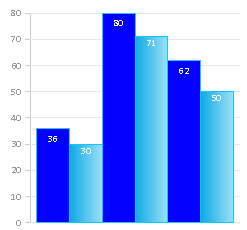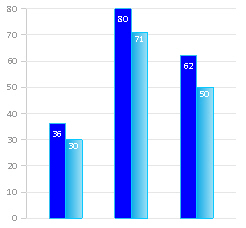This adjacent bar chart shows 100 percent Bar width and 0 percent Bar overlap. This adjacent bar chart shows 50 percent Bar width and 0 percent Bar overlap.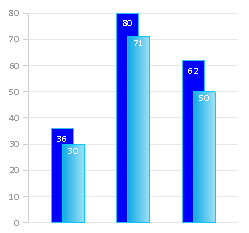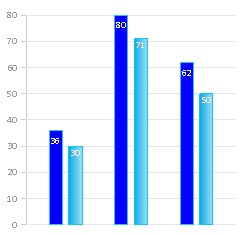This adjacent bar chart shows 50 percent Bar width and 50 percent Bar overlap. This adjacent bar chart shows 50 percent Bar width and -50 percent Bar overlap.

#### Stacked

The Stacked option draws all the variables one on top of the other. The base for additional variables is the ends of the previous variables. The total height of a stacked bar is the sum of all variables (Y values) for each X value.

For example, there are three variables for X with the values 10, 15, and 20. The base of the stacked bar is set to zero. The first bar would be drawn from zero to 10. The next bar would be drawn from 10 to 25, and the final bar would be drawn from 25 to 45.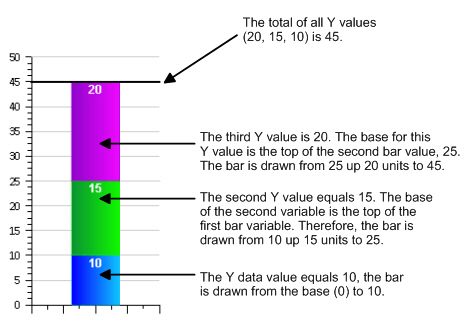This example demonstrates how stacked bar heights are calculated.

### Orientation

Bar charts and floating bar charts can be displayed horizontally or vertically. The Orientation options let you change the orientation of the bars. The default setting is Vertical, which plots the height of the bars vertically. The Horizontal option rotates the bars by 90°, showing the height of the bars horizontally. This results in a bar graph where the bars protrude horizontally from the vertical axis. This setting affects all bar charts in a bar chart group when multiple bar charts are plotted on one graph.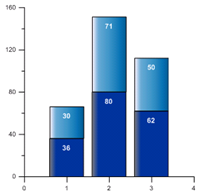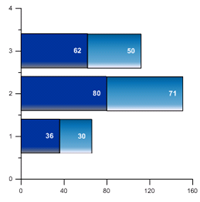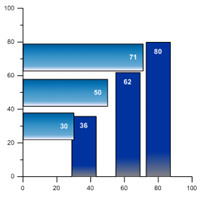This bar chart is displayed vertically. Vertical is the default display. This bar chart is displayed horizontally. These bar charts are in different bar chart groups. One group is horizontal and one is vertical.

### Bar Style

The Bar style option allows the bars to be drawn as rectangles or as another shape. To change the Bar style, click on the existing option. In the list, select a new option. The options available are Box, Cone, Inverted cone, Diamond, Spindle, and Image. The bar chart automatically updates to the new style.

If the Bar style is set to Image, the Set image command is used to load an image to display for the bars. Click the Load button next to Set image to open the Set Image dialog. Select the image and click OK to display the image on the bar chart.

### Slices

In a 3D XYY or 3D XYZ bar chart, when the Bar style is set to an option other than Box, the Slices option becomes available. The Slices option controls the number of sides that the 3D shape has. With a Bar style set to Cylinder, the more Slices the object contains, the closer to a round cylinder the object will be displayed. The Slices option can be set to a whole number between 2 and 100.  When the Slices is set to 2, the object appears as two dimensional. The line properties for the slice lines are set on the Line tab.

### Stacks

In a 3D or 3D XYZ bar chart, when the Bar style is set to an option other than Box, the Stacks option becomes available. The Stacks option controls the number horizontally stacked sections that the 3D shape has. The Stacks option can be set to a whole number between 2 and 100.  The line properties for the stack lines are set on the Line tab.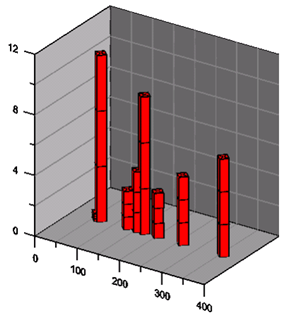This bar chart shows three horizontal stacks for each bar and four slices, making the cylinder diamond shaped.

### Bar Base

The Base and Base value indicate the location on the plot where the bars originate. The graph varies depending on the selected Base value. The Base of the bars can be set to the data minimum, to the data maximum, to zero, or to a custom value. The bars are drawn from the base value to the data point value. This setting affects all bar charts when multiple bar charts are plotted on one graph in the same bar chart group. To change the Base value, click on the existing option next to the Base. Select a new option from the list. Setting the Base to Zero causes all bars to originate at the zero axis value. Positive data values are drawn upward and negative data values are drawn downward. The Data minimum option draws all bars from the data minimum to the data value. The Data maximum option draws all bars from the data maximum to the data value.

The Custom option draws all bars from the Base value to the data value. The bars are drawn upward or downward depending on the data values and the custom base number.

For example, if the Base is set to Zero and the Y data value is 1500, the bar is drawn from zero to 1500 on the Y axis. If the Base is set to Custom and the Base value is set to 5000, the bar is drawn from 5000 to 1500.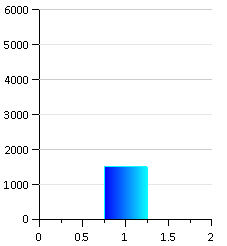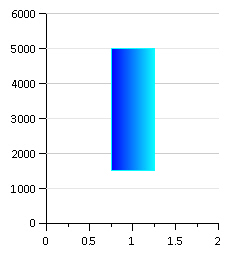The Y value is 1500 and the base is set to 0. The bar is drawn up to 1500. The Y value is 1500 and the base is set to 5000. The bar is drawn down to 1500.

### Width Settings

The Width settings section allows you to select the method to determine the width of the bars.

• When Type is set to Auto, the percentage in the Bar width option determines the width of the bars (from 1 to 50000 percent). A 100 percent width is determined by the range of the X values; the bars touch and cover 100 percent of the data range. When Bar width is set to 50 percent, the bars cover 50 percent of the data range and there are gaps between the bars. When the width is set to a value greater than 100 percent the bars overlap. This setting affects all bar charts when multiple bar charts are plotted on one graph.
• When Type is set to Fixed, the Fixed width option becomes available so you can change the width of all bars in the graph to a fixed width, e.g., 0.25 inches. Enter a value between 0.001 and 50.00 inches (0.003 and 127 cm).
• When Type is set to Variable, the Variable units option becomes available so you can select data from the worksheet to determine the width of the bars. Select from one of the following Variable units options:
• Percentage - select the Percentage variable to set the bar widths. Percentages should be whole numbers. For example, the value 1 in the worksheet column is 1%.
• Page units - select the Page units variable to set the bar widths, for example when you have data that is in inches or centimeters.
• Axis units (width) and Axis units (interval) - use axes units to set bar widths. If you select Axis units (width), select a data column from the Width variable list. If you select Axis units (interval) select a Y2 variable.

NOTE: The values in a selected width variable should be in the same units as the application page units setting. The bar widths will change if the Page units setting is changed in the Options dialog Ruler and Grid page or with the Layout | Display | Units command. Bar widths are still scaled with the Bar width percentage property when the Type is set to Variable.

### Bar Width

The Bar width field controls all of the bars in the graph in the same bar chart group. Set the width of the bars from 1 to 50000 percent. The default width is 80 percent. The 100 percent width is determined by the range of the X values. When the Bar width is set to 100 percent, the bars touch and cover 100 percent of the data range. When the Bar width is set to 50 percent, the bars cover 50 percent of the data range and there are gaps between the bars. When the width is set to a value greater than 100 percent the bars overlap. This setting affects all bar charts when multiple bar charts are plotted on one graph.This graph shows a Bar width of 100%. This is the default setting. This graph shows a Bar width of 50%.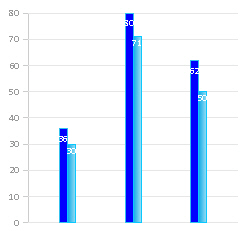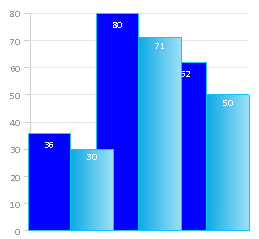This graph shows a Bar width of 25%. This graph shows a Bar width of 125%.

## Axes

Click on the axis name next to the X axis, Y axis, or Z axis fields to change the axes used for the plot. Select another axis name from the list. Alternatively, click Select plots/axis to change the axis used by several plots on a graph at once.

See the Axis - Add to Graph page for information about how to add a new axis to the graph. Once the new axis is added to the graph, it will be available in the X axis, Y axis, or Z axis lists.

## 3D Settings

The 3D Settings section of the Plot page includes the position and width settings for 3D bar charts.

### Position

The Position option controls the location of the plot on the depth axis of the 3D plot. For example, 0 percent places the middle of the plot at the front of the graph, 100 percent places the middle of the plot at the back of the graph, and 50 percent places the plot in the middle of the graph.

### Plot Width

The Plot width controls how wide the plot is displayed. Plots can be 0.01 to 6 inches (0.025 and 15.24 cm) wide.